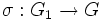# Normal not implies image-potentially fully invariant

This article gives the statement and possibly, proof, of a non-implication relation between two subgroup properties. That is, it states that every subgroup satisfying the first subgroup property (i.e., normal subgroup) need not satisfy the second subgroup property (i.e., image-potentially fully invariant subgroup)
View a complete list of subgroup property non-implications | View a complete list of subgroup property implications
Get more facts about normal subgroup|Get more facts about image-potentially fully invariant subgroup
EXPLORE EXAMPLES YOURSELF: View examples of subgroups satisfying property normal subgroup but not image-potentially fully invariant subgroup|View examples of subgroups satisfying property normal subgroup and image-potentially fully invariant subgroup

## Statement

It is possible to have a normal subgroup$H$ of a group$G$ that is not an image-potentially fully invariant subgroup of$G$, i.e., it is not possible to have a surjective homomorphism$\rho:K \to G$ and a subgroup$L$ of$K$ such that$\rho(L) = H$.

## Proof

Suppose$G$ is the free group of rank two and$H$ is a normal subgroup of$G$ that is not a fully invariant subgroup of$G$. In other words, there exists an endomorphism$\alpha$ of <mah>G[/itex] such that$\alpha(H)$ is not contained inside$H$.

Suppose$\rho:K \to G$ is a surjective homomorphism and$L$ is a subgroup of$K$ such that$\rho(L) = H$. We show that$L$ is not fully invariant in$K$.

Suppose$N$ is the kernel of$\rho$. Since$G$ is a free group,$N$ is a complemented normal subgroup, so there exists a complement$G_1$ to$N$ in$K$ with an isomorphism$\sigma:G_1 \to G$ such that if$\varphi$ is the retraction with kernel$N$ and image$G_1$, then$\rho = \sigma \circ \varphi$. In particular, restricted to$G_1$,$\rho = \sigma$.

Now, consider the endomorphism$\beta$ of$K$ defined as$\beta = \sigma^{-1} \circ \alpha \circ \rho$. Then, we see that$\beta(L) = \sigma^{-1}(\alpha(\rho(L)) = \sigma^{-1}(\alpha(H))$. Thus,$\rho(\beta(L)) = \sigma(\beta(L)) = \alpha(H)$. But$\alpha(H)$ is not contained in$H$, so$\rho(\beta(L))$ is not contained in$H$, so$\beta(L)$ is not contained in$\rho^{-1}(H)$. In particular,$\beta(L)$ is not contained in$L$. Hence,$L$ is not fully invariant in$K$.